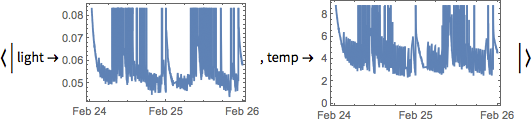# Large Databins

If you're working with multi-megabyte databins, you may want to access only parts.

 In:=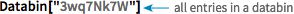X Databin["3wq7Nk7W"]
 Out=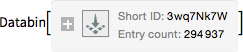In:=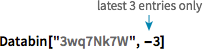X Databin["3wq7Nk7W",-3]
 Out=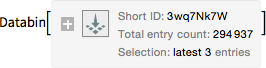In:=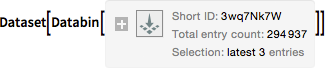X Dataset[Databin["3wq7Nk7W",-3]]
 Out=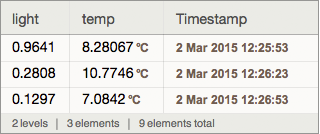Select numbered entries:

 In:=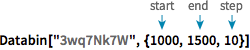X Databin["3wq7Nk7W",{1100,1500,10}]
 Out=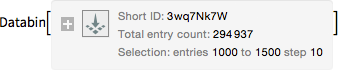In:=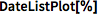X DateListPlot[%]
 Out=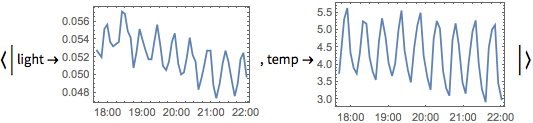Select entries going back a certain amount of time:

("Minute", "Hour", "Day", "Week", "Month", "Year")

 In:=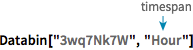X Databin["3wq7Nk7W","Hour"]
 Out=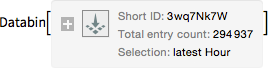In:=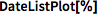X DateListPlot[%]
 Out=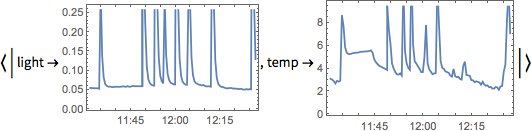Give any timespan: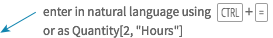In:=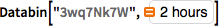X Databin["3wq7Nk7W",Quantity[2,"Hours"]]
 Out=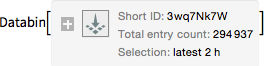In:=X DateListPlot[%]
 Out=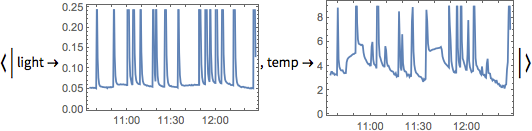Select entries based on date:

 In:=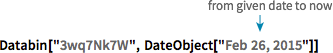X Databin["3wq7Nk7W",DateObject["Feb 26, 2015"]]
 Out=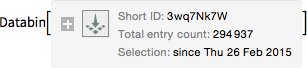In:=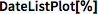X DateListPlot[%]
 Out=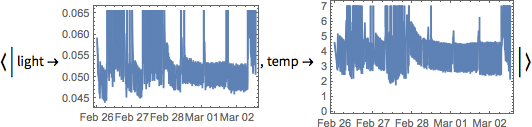Give any timespan:

 In:=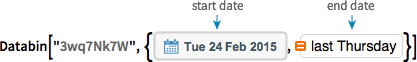X Databin["3wq7Nk7W", {DateObject[{2015, 02, 24}],DateObject[{2015, 02, 26}]}]
 Out=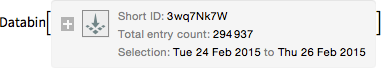In:=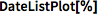X DateListPlot[%]
 Out=